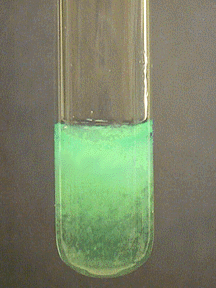# Characteristic Reactions of Nickel Ions (Ni²⁺)

$$\newcommand{\vecs}{\overset { \rightharpoonup} {\mathbf{#1}} }$$ $$\newcommand{\vecd}{\overset{-\!-\!\rightharpoonup}{\vphantom{a}\smash {#1}}}$$$$\newcommand{\id}{\mathrm{id}}$$ $$\newcommand{\Span}{\mathrm{span}}$$ $$\newcommand{\kernel}{\mathrm{null}\,}$$ $$\newcommand{\range}{\mathrm{range}\,}$$ $$\newcommand{\RealPart}{\mathrm{Re}}$$ $$\newcommand{\ImaginaryPart}{\mathrm{Im}}$$ $$\newcommand{\Argument}{\mathrm{Arg}}$$ $$\newcommand{\norm}{\| #1 \|}$$ $$\newcommand{\inner}{\langle #1, #2 \rangle}$$ $$\newcommand{\Span}{\mathrm{span}}$$ $$\newcommand{\id}{\mathrm{id}}$$ $$\newcommand{\Span}{\mathrm{span}}$$ $$\newcommand{\kernel}{\mathrm{null}\,}$$ $$\newcommand{\range}{\mathrm{range}\,}$$ $$\newcommand{\RealPart}{\mathrm{Re}}$$ $$\newcommand{\ImaginaryPart}{\mathrm{Im}}$$ $$\newcommand{\Argument}{\mathrm{Arg}}$$ $$\newcommand{\norm}{\| #1 \|}$$ $$\newcommand{\inner}{\langle #1, #2 \rangle}$$ $$\newcommand{\Span}{\mathrm{span}}$$

• Most common oxidation state: +2
• M.P. 1453º
• B.P. 2732º
• Density 9.91 g/cm3
• Characteristics: Nickel is a silvery-gray metal. Not oxidized by air under ordinary conditions. Easily dissolved in dilute nitric acid.

## Characteristic Reactions of Ni²⁺

Nickel(II) ion forms a large variety of complex ions, such as the green hydrated ion, $$\ce{[Ni(H2O)6]^{2+}}$$.

### Aqueous Ammonia

Aqueous ammonia precipitates green gelatinous Ni(OH)2:

$\ce{Ni^{2+}(aq) + 2NH3(aq) + 2H2O(l) <=> Ni(OH)2(s) + 2NH4^{+}(aq)}$The nickel(II) hydroxide precipitate dissolves in excess ammonia to form a blue complex ion:

$\ce{Ni(OH)2(s) + 6NH3(aq) <=> [Ni(NH3)6]^{2+}(aq) + 2OH^{-}(aq) }$### Sodium Hydroxide

Sodium hydroxide also precipitates nickel(II) hydroxide:

$\ce{Ni^{2+}(aq) + 2OH^{-}(aq) <=> Ni(OH)2(s)}$Nickel(II) hydroxide does not dissolve in excess $$\ce{NaOH}$$.

### Dimethylglyoxime

Addition of an alcoholic solution of dimethylglyoxime to an ammoniacal solution of Ni(II) gives a rose-red precipitate, abbreviated $$\ce{Ni(dmg)2}$$:

$\ce{[Ni(NH3)6]^{2+}(aq) + 2(CH3CNOH)2(alc) <=> Ni[ONC(CH3)C(CH3)NOH]2(s) + 2NH4^{+}(aq) + 4NH3(aq)}$### Sulfide

Black $$\ce{NiS}$$ is precipitated by basic solutions containing sulfide ion:

$\ce{Ni^{2+}(aq) + S2^{-}(aq) <=> NiS(s)}$Nickel(II) sulfide is not precipitated by adding $$\ce{H2S}$$ in an acidic solution. In spite of this, $$\ce{NiS}$$ is only slightly soluble in $$\ce{HCl}$$ and has to be dissolved in hot nitric acid or aqua regia, because $$\ce{NiS}$$ changes to a different crystalline form with different properties.

### No Reaction

$$\ce{Cl^{-}}$$, $$\ce{SO4^{2-}}$$

Characteristic Reactions of Nickel Ions (Ni²⁺) is shared under a not declared license and was authored, remixed, and/or curated by James P. Birk.